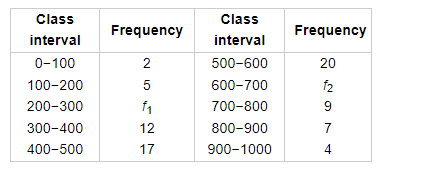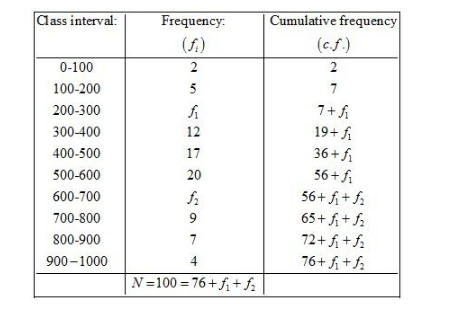# The median of the following data is 525.`
Question:

The median of the following data is 525. Find the missing frequency, if it is given that there are 100 observation in the data:\Solution:

Given: Median = 525

We prepare the cumulative frequency table, as given below.Now, we have

$N=100$

$76+f_{1}+f_{2}=100$

$f_{2}=24-f_{1}$....(1)

So, $\frac{N}{2}=50$

Since median $=525$ so the median class is $500-600$.

Here, $l=500, f=20, F=36+f_{1}$ and $h=100$

We know that

Median $=l+\left\{\frac{\frac{N}{2}-F}{f}\right\} \times h$

$525=500+\left\{\frac{50-\left(36+f_{1}\right)}{20}\right\} \times 100$

$25=\frac{\left(14-f_{1}\right) \times 100}{20}$

$25 \times 20=1400-100 f_{1}$

$100 f_{1}=1400-500$

$f_{1}=\frac{900}{100}$

$=9$

Putting the value of $f_{1}$ in (1), we get

$f_{2}=24-9$

$=15$

Hence, the missing frequencies are 9 and 15.